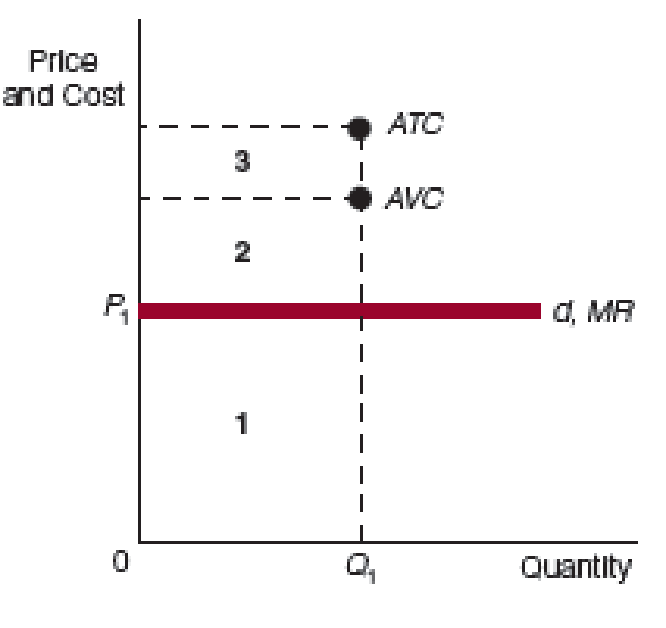Chapter 22, Problem 8WNG

Chapter
Section
Textbook Problem

In the accompanying figure, what area(s) represent(s) the following at Q1? a. Total cost b. Total variable cost c. Total revenue d. Loss (negative profit)(a)

To determine

Identity the area of total cost in the figure.

Explanation

Figure 1 shows the area of AVC, ATC, and MR.

In Figure 1, the vertical axis measures price and cost. The horizontal axis measures quantity...

(b)

To determine

Identity the area of total variable cost in the figure.

(c)

To determine

Identity the area of total revenue in the figure.

(d)

To determine

Identity the area of loss in the figure.

Still sussing out bartleby?

Check out a sample textbook solution.

See a sample solution

The Solution to Your Study Problems

Bartleby provides explanations to thousands of textbook problems written by our experts, many with advanced degrees!

Get Started

Some income from capital is taxed twice. Explain.

Brief Principles of Macroeconomics (MindTap Course List)

What is cultural diversity in an organization?

Foundations of Business (MindTap Course List)

If a firms earnings per share grew from 1 to 2 over a 10-year period, the total growth would be 100%, but the a...

Fundamentals of Financial Management, Concise Edition (with Thomson ONE - Business School Edition, 1 term (6 months) Printed Access Card) (MindTap Course List)